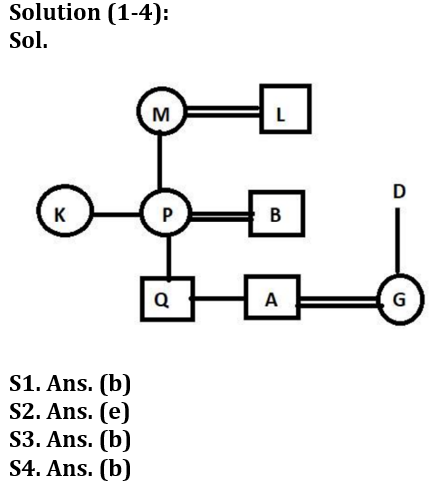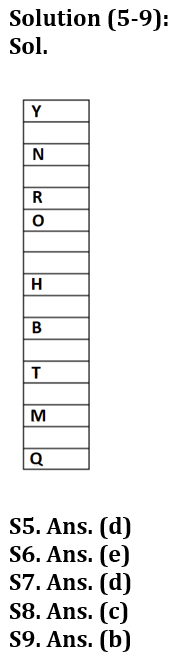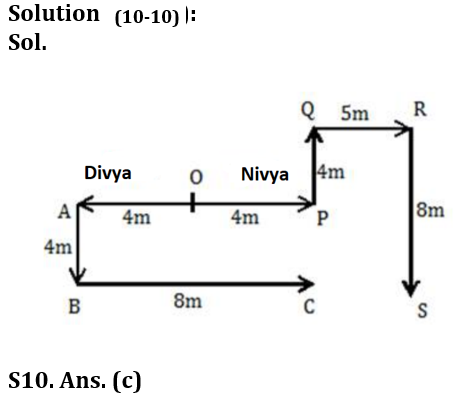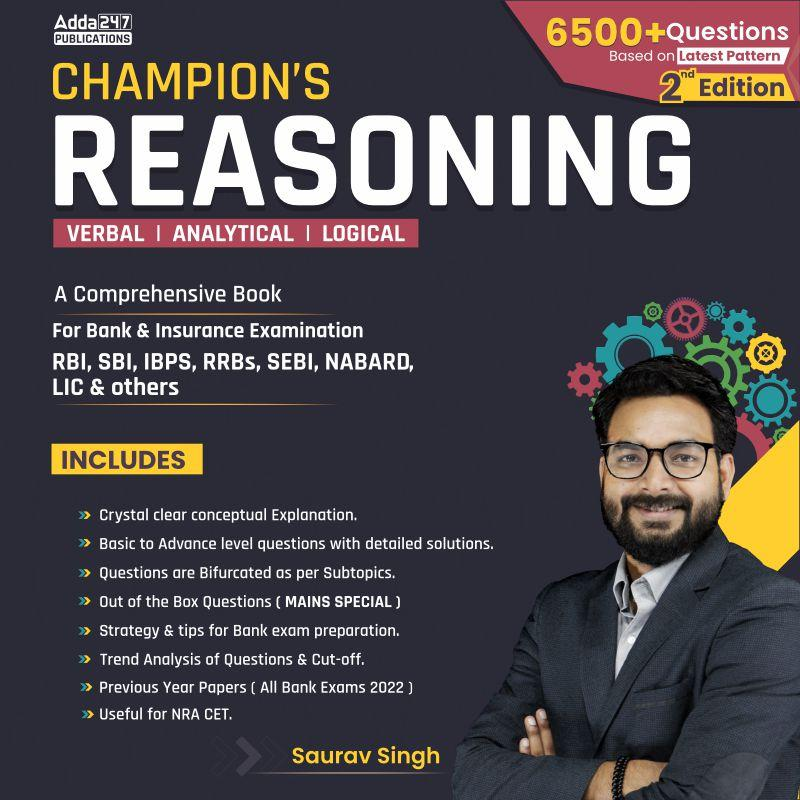Latest Banking jobs   »   BANK FOUNDATION reasoning

# Reasoning Quiz For Bank Foundation 2023 -17th September

Direction (1-4): Read the following information carefully and answer the questions given below.
In a family of three-generation, there are nine members and three married couples. M has no son. P who is the only married child of M is married to B. Both M and P have two children. Q is not a female member of the family. A is the son-in-law of D. L is the father of K and father-in-law of B. G is the sister-in-law of Q. M is grandmother of Q.
Q1. What is the relation of G with respect to P?
(a) Son-in-law
(b) Daughter-in-law
(c) Son
(d) Daughter
(e) None of these
Q2. How many female members are there in the family?
(a) Two
(b) Three
(c) One
(d) Four
(e) Cannot be determined
Q3. Who is the grandson of L?
(a) A
(b) Both (a) and (d)
(c) D
(d) Q
(e) No one
Q4. What is the relation of A with respect to K?
(a) Son
(b) Nephew
(c) Niece
(d) Father
(e) Cannot be determined
Direction (5-9): Study the following information carefully and answer the questions given below.
A certain number of boxes are placed one above the other in a stack. Box H is placed two places above box B. Three boxes are placed between B and M. M is not placed above B. Not more than one box is placed between M and Q. Minimum four boxes are placed between B and Q. The number of boxes between H and N is the same as the number of boxes between H and M. O is placed just below R. Four boxes are placed between Y and O in which Y is placed above N. One box can be placed between Y and N. T is placed exactly between B and M. At least three boxes are placed between T and Q. Not more than two boxes are placed between H and O.
Q5. Which of the following box is placed at bottom?
(a) R
(b) N
(c) O
(d) Q
(e) Y
Q6. How many boxes are placed between Y and T?
(a) Two
(b) Three
(c) Five
(d) Six
(e) None of these

Q7. How many boxes placed between N and R?
(a) Five
(b) Two
(c) Three
(d) One
(e) None of these
Q8. How many total boxes are placed in this stack?
(a) Fifteen
(b) Sixteen
(c) Seventeen
(d) Eighteen
(e) None of these
Q9. If U is placed at sixth position below Q, then how many boxes are placed between O and U?
(a) Fifteen
(b) Sixteen
(c) Seventeen
(d) Eighteen
(e) None of these
Direction (10-10): Read the following information carefully to answer the questions based on it.
Nivya and Divya start their journey from the same point ‘O’. Nivya walks 4 meters to the east and reaches point P. Then she turns to her left and walks 4 meters and reaches point Q. Then she turns to her right and walks 5 meters and reaches point R. Finally, she turns to her right and walks 8 meters and reached point S. On the other hand, Divya walks 4 meters to the west and reaches point A. Then she turns to her left and walks 4 meters and reaches point B. Finally, she turns to her left end walks 8 meters and reached point C.
Q10. What is the minimum distance between point C and point S?
(a) 8m
(b) 3m
(c) 5m
(d) 4m
(e) None of these

Solutions## FAQs

### What is the selection process of the Bank Clerk?

The selection process of the Bank Clerk is Prelims & Mains.

#### Congratulations!Union Budget 2023-24: Free PDF